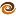# EmptyCrate

#### About Me | Contact Me | Training |ChaiScript.com | YouTube Channel

We’ve covered the “Assembly Language”, “C” and “C++” of the C++ threading world, and now we are going to try and move beyond that.

In the “C++” example we showed a object that automatically managed a thread’s life time and used the thread to calculate the Fibonacci sequence in the background. Using templates we should be able to make a generic version of the Fibonacci calculator thread. We will not go into all of the implementation details here, but will instead focus on the possibilities.

Future
A future is a method of letting a value calculate in the background and doing a block-wait for it when you want the value.

``````future<int> Fib1 = boost::bind(&calculatefib, 1);
future<int> Fib2 = boost::bind(&calculatefib, 2);
future<int> Fib3 = boost::bind(&calculatefib, 3);
``````

Three threads were created in the above example and each began calculating its respective Fibonacci value. If the user were to try and access a future value the application would either return the value immediately if it were already available or block until it became available.

``````std::cout << Fib1 << std::endl; // Return immediately if the value is ready or wait for it to become ready
``````

Worker
A worker performs work in the background, without handling a return value and cleans itself up when it goes out of scope.

``````vector parallelfibcalculator()
{
vector<int> values(4);
worker w0 = boost::bind(&calculatefib, 0, boost::ref(values));
worker w1 = boost::bind(&calculatefib, 1, boost::ref(values));
worker w2 = boost::bind(&calculatefib, 2, boost::ref(values));
worker w3 = boost::bind(&calculatefib, 3, boost::ref(values));
return values; // when w3-w0 try to destruct they will block until work is finished
}
``````

Calling `parallelfibcalculator()` will spawn four threads for calculating the first four Fibonacci values then block until all four are completed.

These two examples are just a look at the possibilities. With a little creativity it is possible to come up with all kinds of automatic thread handlers that are suited to specific domain needs and simplify the use and management of threads.

My Videos

Related